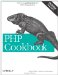# Recipe 2.16. Calculating Using Numbers in Bases Other Than Decimal

#### 2.16.1. Problem

You want to perform mathematical operations with numbers formatted not in decimal, but in octal or hexadecimal. For example, you want to calculate web-safe colors in hexadecimal.

#### 2.16.2. Solution

Prefix the number with a leading symbol, so PHP knows it isn't in base 10. The following values are all equal:

`0144  // base 8  100  // base 10 0x64  // base 16`

Here's how to count from decimal 1 to 15 using hexadecimal notation:

`for (\$i = 0x1; \$i < 0x10; \$i++) { print "\$i\n"; }`

#### 2.16.3. Discussion

Even if you use hexadecimally formatted numbers in a for loop, by default all numbers are printed in decimal. In other words, the code in the Solution doesn't print out "..., 8, 9, a, b, ...." To print in hexadecimal, use one of the methods listed in Recipe 2.15. Here's an example:

`for (\$i = 0x1; \$i < 0x10; \$i++) { print dechex(\$i) . "\n"; }`

For most calculations, it's easier to use decimal. Sometimes, however, it's more logical to switch to another base'for example, when using the 216 web-safe colors. Every web color code is of the form RRGGBB, where RR is the red color, GG is the green color, and BB is the blue color. Each color is actually a two-digit hexadecimal number between 0 and FF.

What makes web-safe colors special is that RR, GG, and BB each must be one of the following six numbers: 00, 33, 66, 99, CC, or FF (in decimal: 0, 51, 102, 153, 204, or 255). So 003366 is web safe, but 112233 is not. Web-safe colors render without dithering on a 256-color display.

When creating a list of these numbers, use hexadecimal notation in this triple-loop to reinforce the list's hexadecimal basis, as shown in Example 2-9.

##### Printing out all the web-safe color codes

 ``

Here the loops compute all possible web-safe colors. However, instead of stepping through them in decimal, you use hexadecimal notation, because it reinforces the hexadecimal link between the numbers. Print them out using printf( ) to format them as uppercase hexadecimal numbers at least two digits long. One-digit numbers are printed with a leading zero.

Recipe 2.15 for details on converting between bases; Chapter 3, "Web Design Principles for Print Designers," in Web Design in a Nutshell by Jennifer Niederst Robbins (O'Reilly) .PHP Cookbook: Solutions and Examples for PHP Programmers
ISBN: 0596101015
EAN: 2147483647
Year: 2006
Pages: 445

Similar book on Amazon